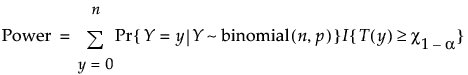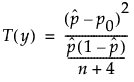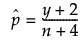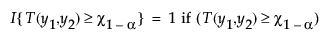Design of Experiments Guide > Prospective Sample Size and Power > One Sample Proportion Calculator > Statistical Details for the One Proportion Calculator
Publication date: 04/12/2021

## Statistical Details for the One Proportion Calculator

The one proportion sample size computations use exact methods based on the binomial distribution. Exact calculations guarantee that the stated power level is obtained.

### Agresti-Coull Method

The exact Agresti-Coull method uses the adjusted Wald-based test statistic. JMP calculates power under the two-sided null hypothesis as follows:where:and 0 otherwise, and

χ1-α is the (1 - α)thquantile of the χ12 distribution.

Because there is not a closed-form expression for n or p0, numerical techniques are used to solve for n or p0.

For more information about the adjusted Wald test statistic, see Agresti and Coull (1998). For more information about calculations in JMP, see Barker (2011, Section 3.3).

### Clopper-Pearson Method

The exact Clopper-Pearson method is based on the binomial distribution. This method results in an alpha level equal to or greater than the stated level. The Clopper-Pearson method is more conservative (larger sample size) than the Agresti-Coull method.

The exact Clopper-Pearson method uses the binomial distribution directly. Numerical techniques are used to solve for the unknown parameter.

For more information about the Clopper-Pearson exact method, see Clopper and Pearson (1934) or Agresti and Coull (1998, Section 1).

Want more information? Have questions? Get answers in the JMP User Community (community.jmp.com).